Circle

Chapter 11 Class 11 Conic Sections
Concept wise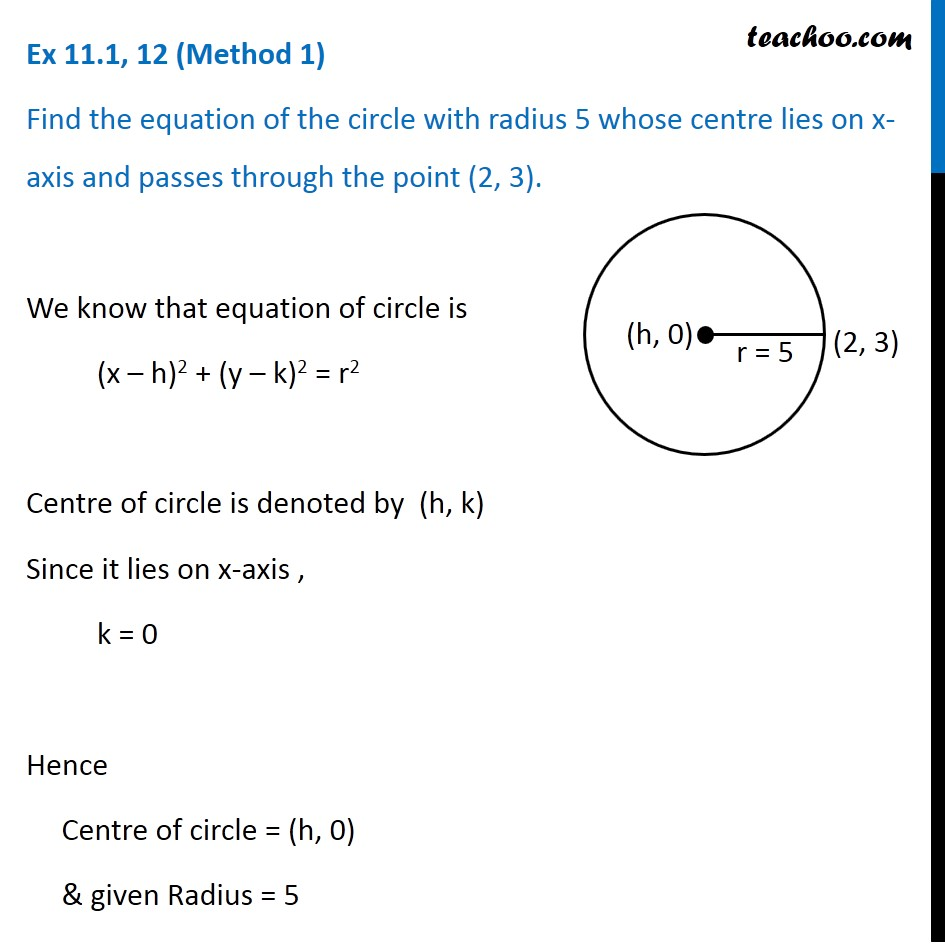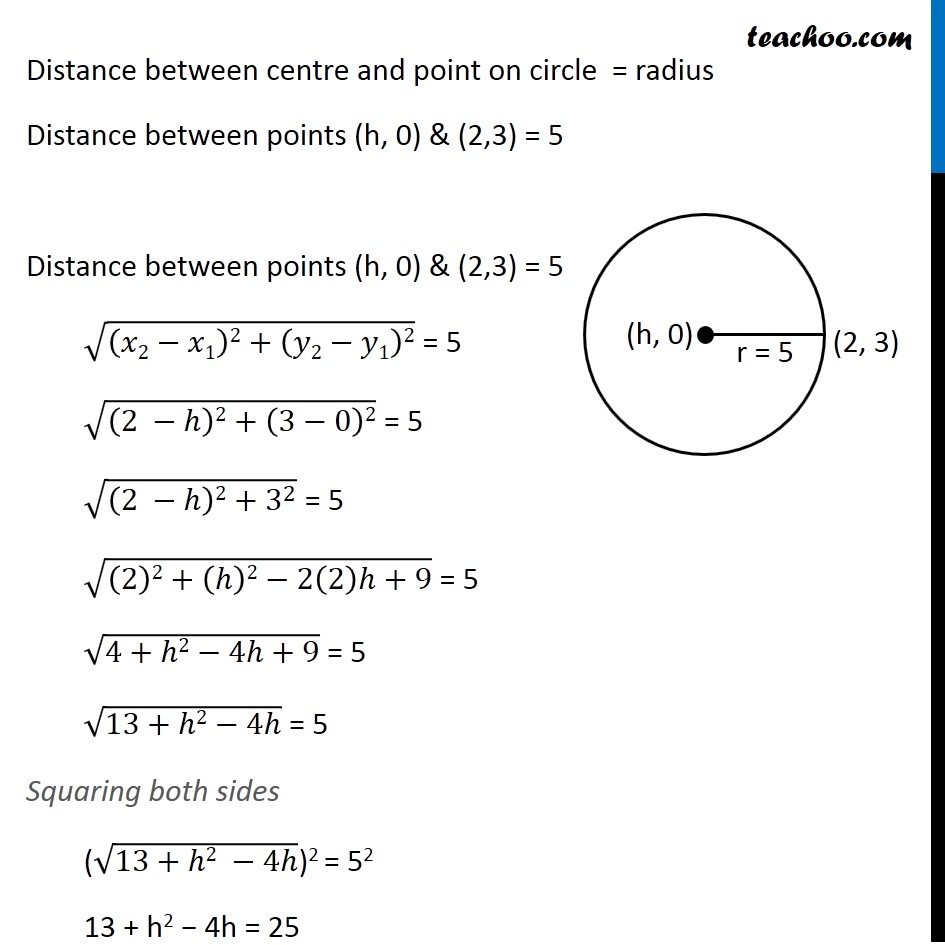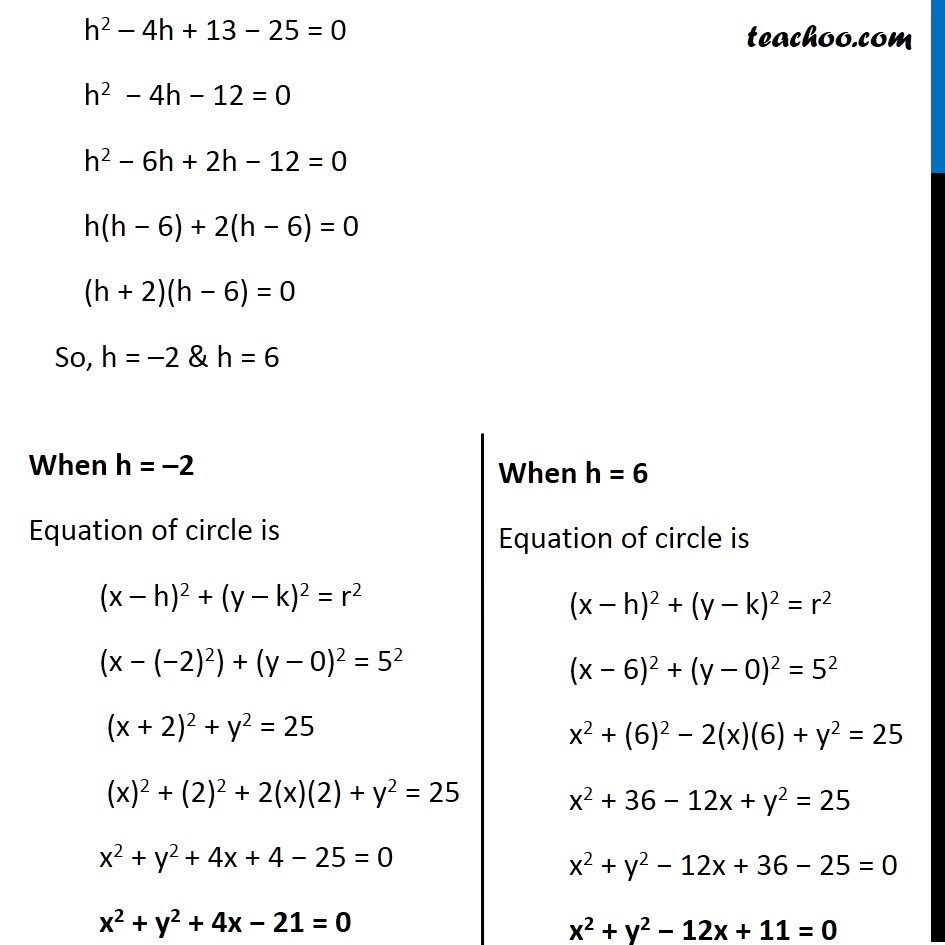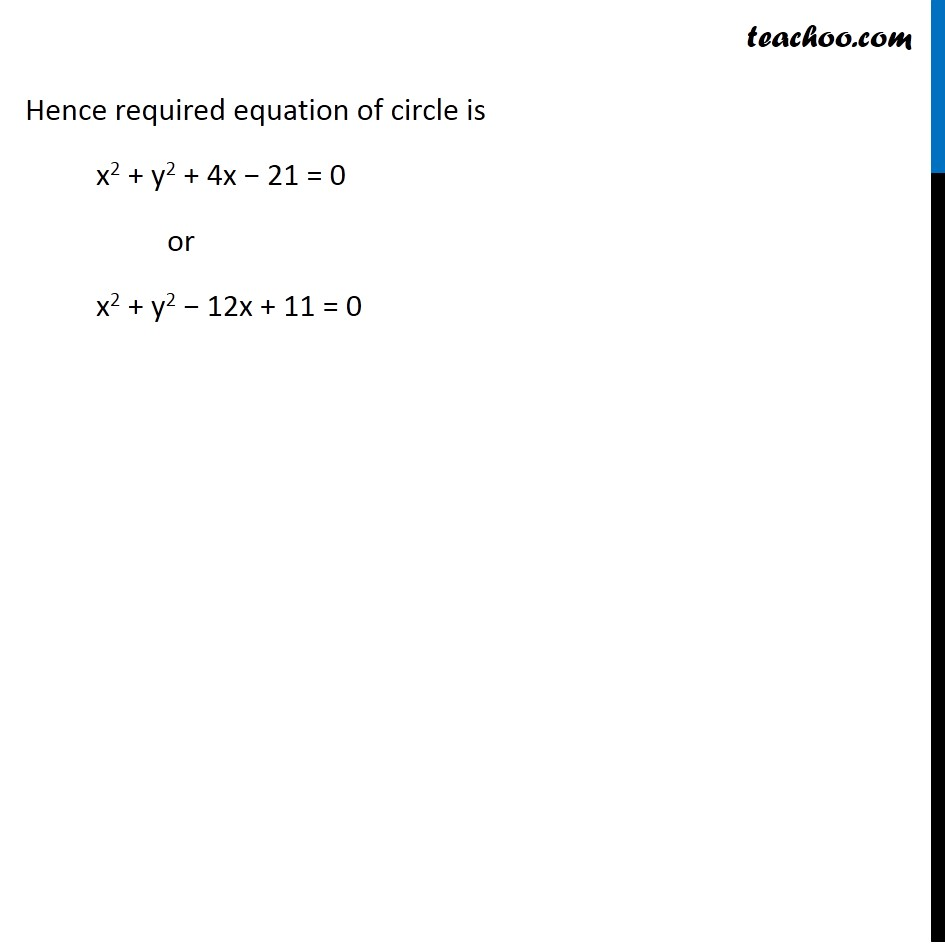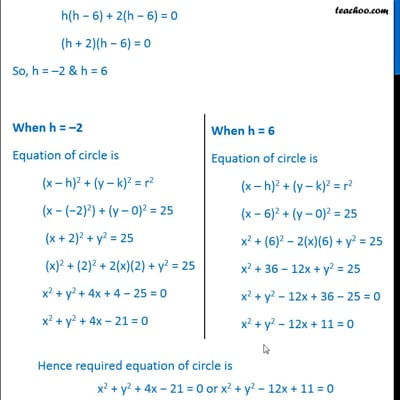This video is only available for Teachoo black users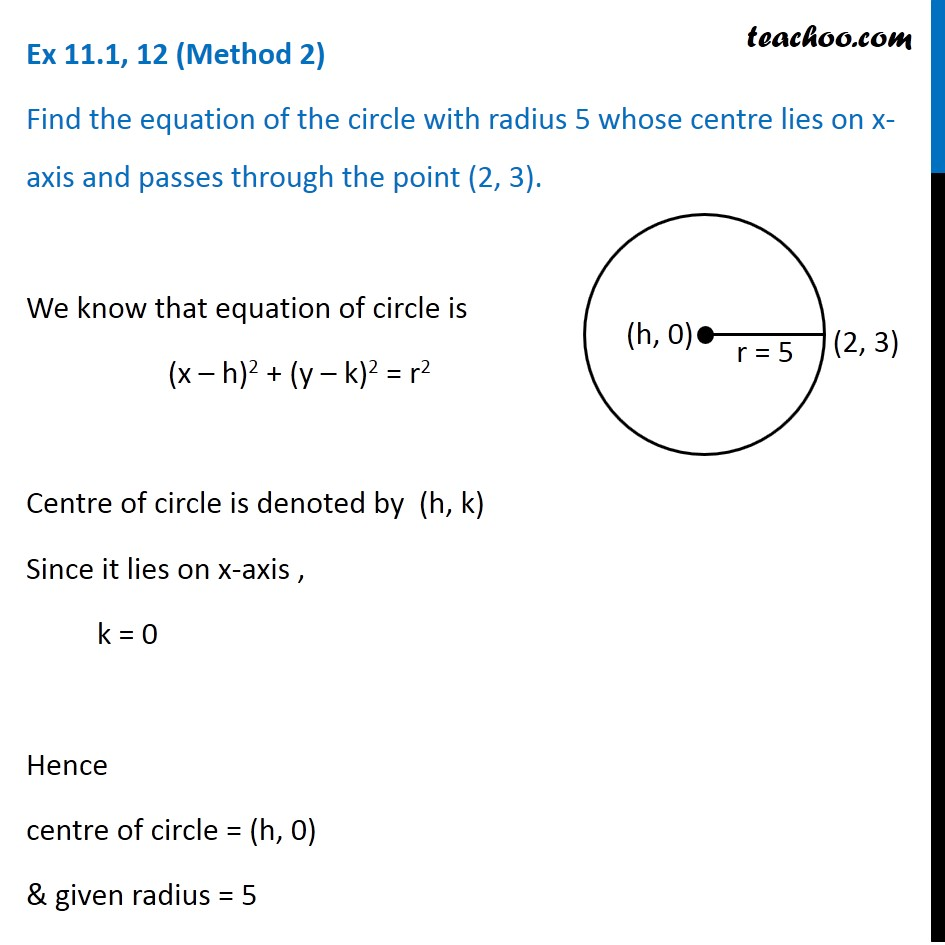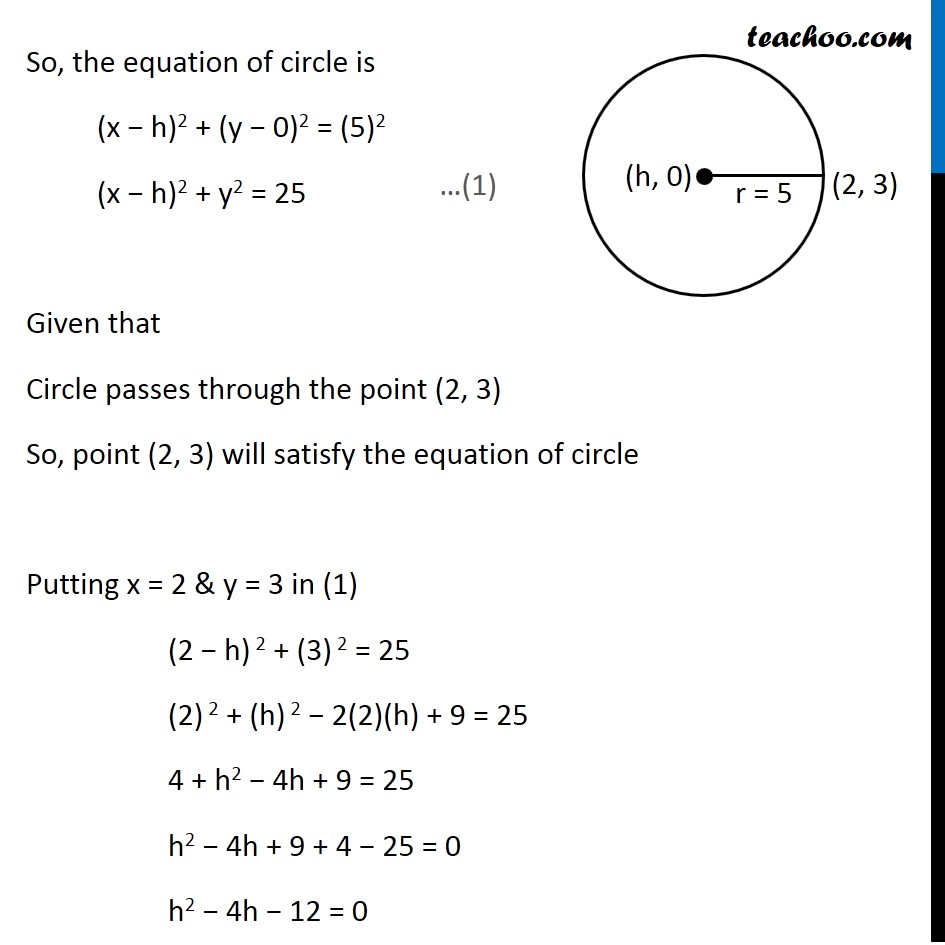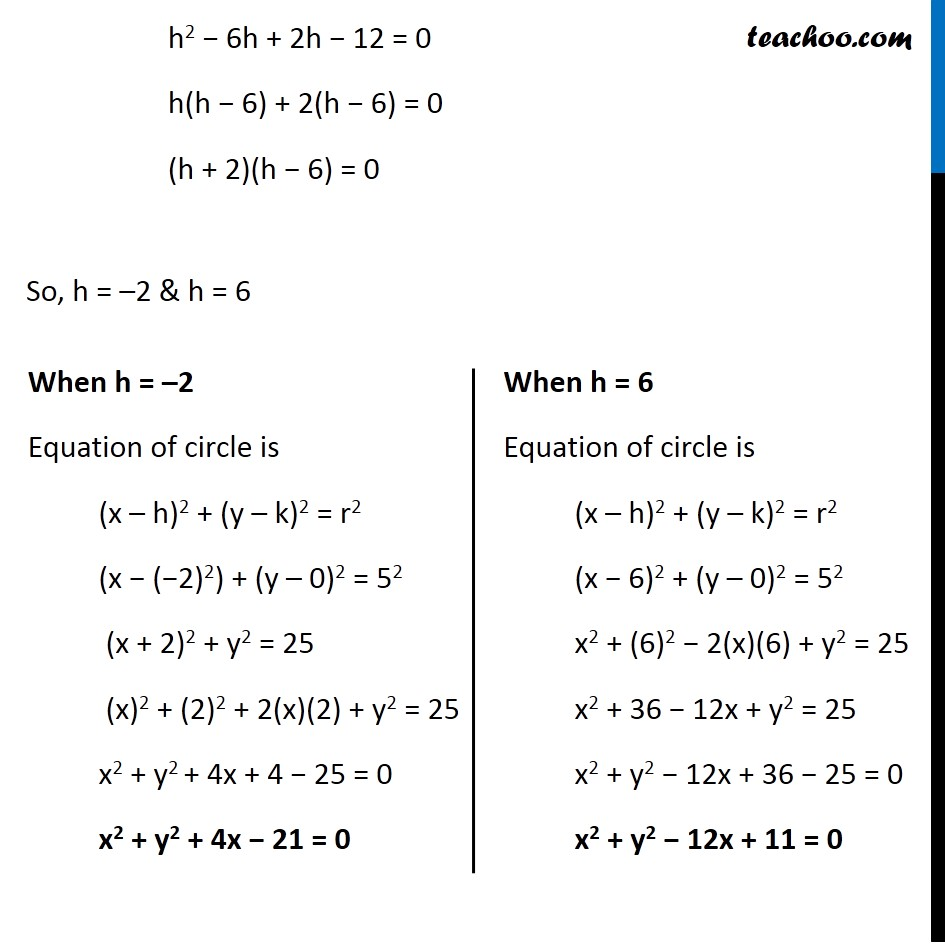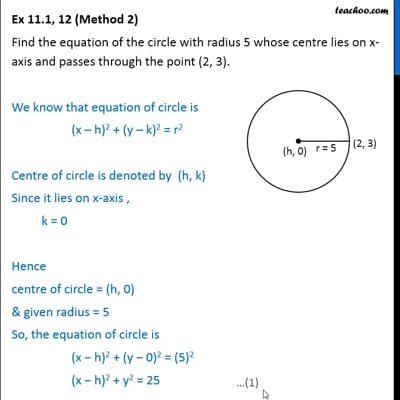This video is only available for Teachoo black users

Introducing your new favourite teacher - Teachoo Black, at only ₹83 per month

### Transcript

Ex 11.1, 12 (Method 1) Find the equation of the circle with radius 5 whose centre lies on x-axis and passes through the point (2, 3). We know that equation of circle is (x – h)2 + (y – k)2 = r2 Centre of circle is denoted by (h, k) Since it lies on x-axis , k = 0 Hence Centre of circle = (h, 0) & given Radius = 5 Distance between centre and point on circle = radius Distance between points (h, 0) & (2,3) = 5 Distance between points (h, 0) & (2,3) = 5 √((𝑥2−𝑥1)2+(𝑦2−𝑦1)2) = 5 √((2 −ℎ)2+(3−0)2) = 5 √((2 −ℎ)2+3^2 ) = 5 √((2)2+(ℎ)2−2(2)ℎ+9) = 5 √(4+ℎ2−4ℎ+9) = 5 √(13+ℎ2−4ℎ) = 5 Squaring both sides (√(13+ℎ2 −4ℎ))2 = 52 13 + h2 − 4h = 25 h2 – 4h + 13 − 25 = 0 h2 − 4h − 12 = 0 h2 − 6h + 2h − 12 = 0 h(h − 6) + 2(h − 6) = 0 (h + 2)(h − 6) = 0 So, h = –2 & h = 6 When h = –2 Equation of circle is (x – h)2 + (y – k)2 = r2 (x − (−2)2) + (y – 0)2 = 52 (x + 2)2 + y2 = 25 (x)2 + (2)2 + 2(x)(2) + y2 = 25 x2 + y2 + 4x + 4 − 25 = 0 x2 + y2 + 4x − 21 = 0 When h = 6 Equation of circle is (x – h)2 + (y – k)2 = r2 (x − 6)2 + (y – 0)2 = 52 x2 + (6)2 − 2(x)(6) + y2 = 25 x2 + 36 − 12x + y2 = 25 x2 + y2 − 12x + 36 − 25 = 0 x2 + y2 − 12x + 11 = 0 Hence required equation of circle is x2 + y2 + 4x − 21 = 0 or x2 + y2 − 12x + 11 = 0 Ex 11.1, 12 (Method 2) Find the equation of the circle with radius 5 whose centre lies on x-axis and passes through the point (2, 3). We know that equation of circle is (x – h)2 + (y – k)2 = r2 Centre of circle is denoted by (h, k) Since it lies on x-axis , k = 0 Hence centre of circle = (h, 0) & given radius = 5 So, the equation of circle is (x − h)2 + (y − 0)2 = (5)2 (x − h)2 + y2 = 25 Given that Circle passes through the point (2, 3) So, point (2, 3) will satisfy the equation of circle Putting x = 2 & y = 3 in (1) (2 − h) 2 + (3) 2 = 25 (2) 2 + (h) 2 − 2(2)(h) + 9 = 25 4 + h2 − 4h + 9 = 25 h2 − 4h + 9 + 4 − 25 = 0 h2 − 4h − 12 = 0 h2 − 6h + 2h − 12 = 0 h(h − 6) + 2(h − 6) = 0 (h + 2)(h − 6) = 0 So, h = –2 & h = 6 When h = –2 Equation of circle is (x – h)2 + (y – k)2 = r2 (x − (−2)2) + (y – 0)2 = 52 (x + 2)2 + y2 = 25 (x)2 + (2)2 + 2(x)(2) + y2 = 25 x2 + y2 + 4x + 4 − 25 = 0 x2 + y2 + 4x − 21 = 0 When h = 6 Equation of circle is (x – h)2 + (y – k)2 = r2 (x − 6)2 + (y – 0)2 = 52 x2 + (6)2 − 2(x)(6) + y2 = 25 x2 + 36 − 12x + y2 = 25 x2 + y2 − 12x + 36 − 25 = 0 x2 + y2 − 12x + 11 = 0 Hence required equation of circle is x2 + y2 + 4x − 21 = 0 or x2 + y2 − 12x + 11 = 0